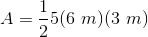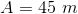# High School Math : How to find the area of a pentagon

## Example Questions

### Example Question #1 : How To Find The Area Of A Pentagon

What is the area of a regular pentagon with side length?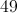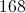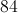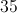Explanation:

The area of a regular polygon can be solved using the following equation: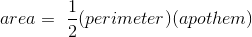.

To find the perimeterof the pentagon, we multiply the side length by five.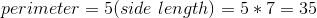The apothem of a regular polygon (= number of sides;= side length) is given by the equation below.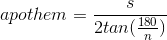Pluggin in our numbers, we can solve for the apothem.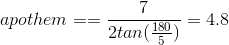Finally, we can solve for the area.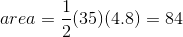### Example Question #2 : How To Find The Area Of A Pentagon

Find the area of the following pentagon: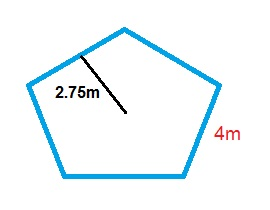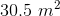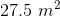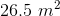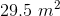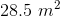Explanation:

The formula for the area of of a pentagon is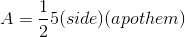.

Plugging in our values, we get: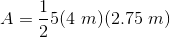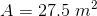### Example Question #3 : How To Find The Area Of A Pentagon

Find the area of the following pentagon: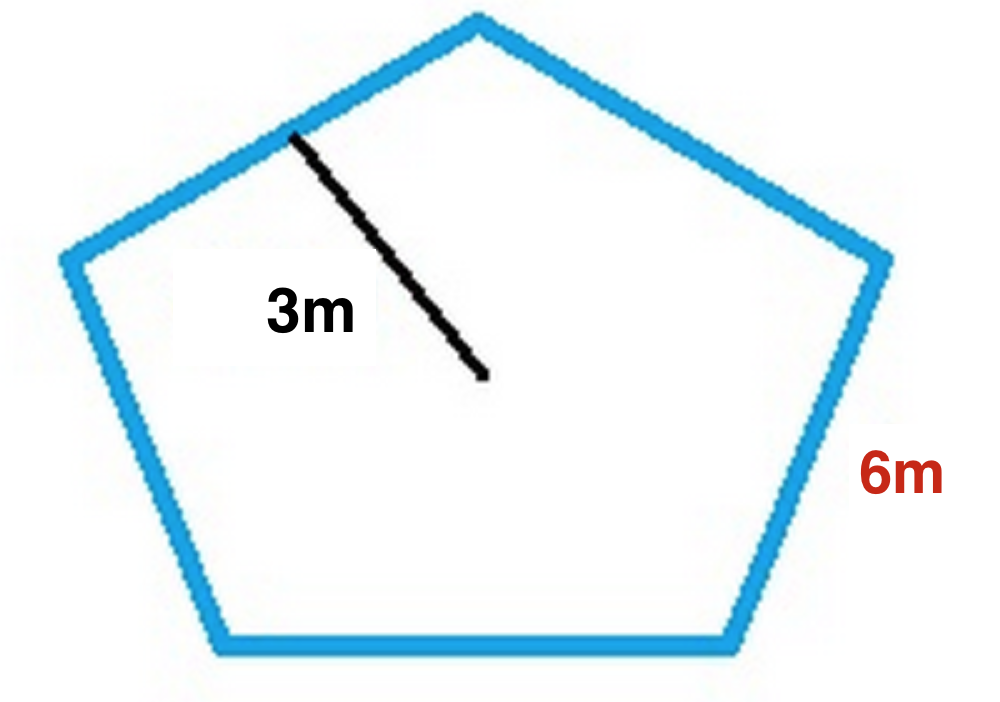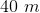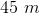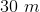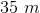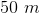Explanation:

The formula for the area of of a pentagon is:Plugging in our values, we get: